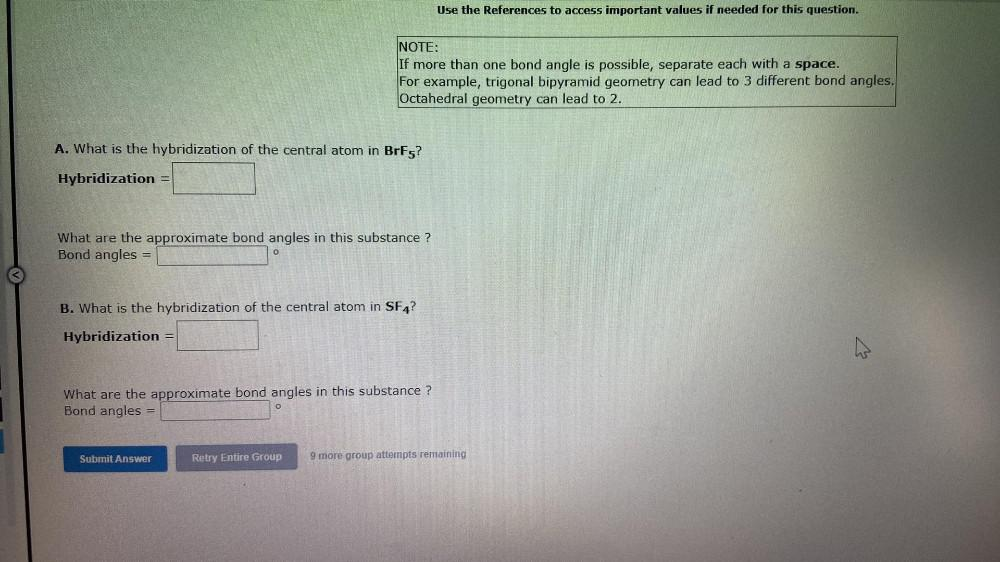Question:

# Use the References to access important values if needed for this question. NOTE: If more than one bond angle is possible, separaUse the References to access important values if needed for this question. NOTE: If more than one bond angle is possible, separate each with a space. For example, trigonal bipyramid geometry can lead to 3 different bond angles. Octahedral geometry can lead to 2. A. What is the hybridization of the central atom in BrFg? Hybridization What are the approximate bond angles in this substance ? Bond angles B. What is the hybridization of the central atom in SF4? Hybridization w What are the approximate bond angles in this substance ? Bond angles Submit Answer Retry Entire Group 9 more group attempts remaining